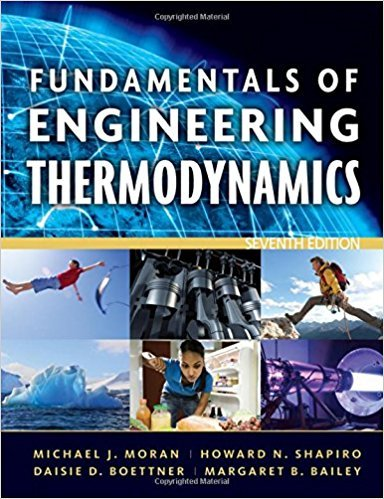×
Get Full Access to Fundamentals Of Engineering Thermodynamics - 7 Edition - Chapter 3 - Problem 79p
Get Full Access to Fundamentals Of Engineering Thermodynamics - 7 Edition - Chapter 3 - Problem 79p

×

# Applying the Energy BalanceOne-half kg of Refrigerant-22ISBN: 9780470495902 50

## Solution for problem 79P Chapter 3

Fundamentals of Engineering Thermodynamics | 7th Edition

• Textbook Solutions
• 2901 Step-by-step solutions solved by professors and subject experts
• Get 24/7 help from StudySoup virtual teaching assistantsFundamentals of Engineering Thermodynamics | 7th Edition

4 5 1 259 Reviews
21
2
Problem 79P

Problem 79P

Applying the Energy Balance

One-half kg of Refrigerant-22 is contained in a piston-cylinder assembly, initially saturated vapor at 5 bar. The refrigerant undergoes a process for which the pressure-specific volume relation is pv = constant to a final pressure of 20 bar. Kinetic and potential energy effects can be neglected. Determine the work and heat transfer for the process, each in kJ.

Step-by-Step Solution:

Solution

Step 1 of 3

Consider aof Refrigerantis contained in a piston-cylinder assembly, initially saturated at. The pressure-specific volume relation isin which the refrigerant undergoes a process , we need to find out the work and the heat transfer for the process.

Step 2 of 3

Step 3 of 3

##### ISBN: 9780470495902

The full step-by-step solution to problem: 79P from chapter: 3 was answered by , our top Engineering and Tech solution expert on 07/20/17, 09:01AM. The answer to “Applying the Energy BalanceOne-half kg of Refrigerant-22 is contained in a piston-cylinder assembly, initially saturated vapor at 5 bar. The refrigerant undergoes a process for which the pressure-specific volume relation is pv = constant to a final pressure of 20 bar. Kinetic and potential energy effects can be neglected. Determine the work and heat transfer for the process, each in kJ.” is broken down into a number of easy to follow steps, and 61 words. This textbook survival guide was created for the textbook: Fundamentals of Engineering Thermodynamics, edition: 7. This full solution covers the following key subjects: bar, refrigerant, process, pressure, Energy. This expansive textbook survival guide covers 14 chapters, and 1501 solutions. Since the solution to 79P from 3 chapter was answered, more than 502 students have viewed the full step-by-step answer. Fundamentals of Engineering Thermodynamics was written by and is associated to the ISBN: 9780470495902.

Unlock Textbook Solution